# Modeling Malthusian Dynamics in Pre-industrial Societies

Author: Nefedov, Sergey
Journal: Social Evolution & History. Volume 13, Number 1 / March 2014

Sergey A. Nefedov
Ural Federal University, Ekaterinburg

ABSTRACT

The discussion about the Malthusian character of pre-industrial economies that has arisen in the recent years extensively uses simple mathematical models. This article analyzes some of these models to determine their conformity with Malthusian postulates. The author suggests two models that are more adequate for the description of the Malthusian patterns.

Until recently, most economic historians were inclined to think that the medieval economies in Eurasia had a Malthusian nature (Allen 2008: 951). However, following the publication of Lee and Anderson's work (Lee and Anderson 2002), many came to dispute this opinion. A discussion has arisen about how the available data confirm the Malthusian relationship between demographic dynamics and consumption (i.e., real wages). This discussion has largely involved simple mathematical models of the Malthusian economy.

In 1980, Lee published the first and most popular of these models. This model describes the relationship between the real wage, wt (consumption), and labor resources, Nt (population), using the following equation: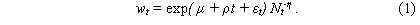Or, in the logarithmic form: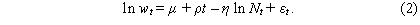Here, t is time; μ, ρ, η are some non-negative constants; and εt is a variable that takes into account the climatic effect and other exogenous parameters. The factor ρ describes the capital increase and technological advances, thus the Malthusian economy features ρ = 0. If we take into account that in the ideal case εt = 0, the model can be expressed with quite a simple equation: wt = С Nt-η, where С is a certain constant. The drawbacks of this equation are evident: a small population Nt results in a consumption rate close to infinity, while in a large population the consumption becomes too small to ensure subsistence. Besides, this equation only shows the relationship between population and consumption. The model contains no feedback to demonstrate in what way the consumption influences population growth.

Wood (1998) suggests a feedback option for which he derives an equation basing on the same equation (1) as Lee, but formulates it as follows: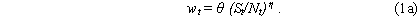Here, θ is the minimum per capita consumption rate, and St is the maximum population that can subsist in the given territory when the consumption equals θ. St can grow with technological advances, but the Malthusian case features a constant St, St = S0. Wood believes that the birth rate bt and death rate dt can be described with the following equations:

where β0 , β1 , β2, δ0, δ1, and δ2 are certain constants. Thus, the equation below describes population growth: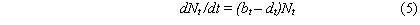Deriving bt and dt from the system of Equations (3)–(4) and inserting them into (5) yields: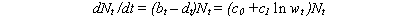where с0 and с1 are certain constants. Substituting equation (1a) here produces: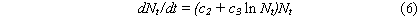where с2 and с3 are certain constants. The differential equation (6) has the time-independent solution Nt = N0 = exp(–с23); its chart will be a horizontal line. According to the theorem of the unique existence of the solution, no other solutions (integral curves) may cross this horizontal line. The derivative dNt /dt is positive below this line, in the area 0 < Nt < N0; the solutions monotonically increase and the integral curves approximate the horizontal line. The derivative dNt /dt is negative above this line; the solutions monotonically decrease and the integral curves approximate the horizontal line from above. Finally, the solutions cannot oscillate: the population cannot first feature growth and then loss due to ‘the Malthusian crises’. Wood justifies this behavior of his model stating that the Malthusian crises ‘are not a necessary feature of Malthusian systems… This conclusion is contrary to the belief of many economic historians (e.g., Le Roy Ladurie 1974: passim; Postan and Hatcher 1985: 69) though not to anything that Malthus himself ever wrote’ (Wood 1998: 110).

However, Malthus did write about population loss and depopulation:

The power of population is so superior to the power of the earth to produce subsistence for man that premature death must in some shape or other visit the human race. The vices of mankind are active and able ministers of depopulation. They are the precursors in the great army of destruction, and often finish the dreadful work themselves. But should they fail in this war of extermination, sickly seasons, epidemics, pestilence, and plague advance in terrific array, and sweep off their thousands and tens of thousands. Should success be still incomplete, gigantic inevitable famine stalks in the rear, and with one mighty blow levels the population with the food of the world (Malthus 1798: 61).

Wood's model does not, therefore, describe the population dynamics envisioned by Malthus himself. Nevertheless, it is used in many studies devoted to the analysis of the Malthusian economy in traditional societies.

Sometimes an iterative version of this model is used, implying calculations on an annual basis. Equation (1a) in the version put forth by Møller and Sharp (2009) has logarithmic form:

Birth and death rates are calculated from the simplified equations: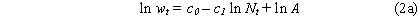The population Nt is related to the population Nt-1 in the previous year through the following relationship: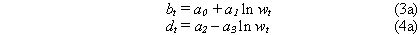here, A, c0, c1 , a0 … a3 are certain constants. Inserting (3a) and (4a) into (7) gives: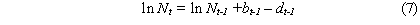where u and v are certain constants. The resulting equation is: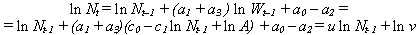This equation generates a series of population values. If the population at the initial moment equals a million (i.e., N1 =1), then N2 = v, N3 equals v raised to a power of 1+u; N3 equals to v raised to a power of 1 + u + u2, etc. If ׀u ׀> 1, then Nt → ∞, which is impossible under the condition of limited resources in the Malthusian theory. If 0 < u < 1, then Nt monotonically tends to a finite bound. Finally, the case –1 < u <0 produces a very specific series in which the population increases in even years and decreases in odd years (or vice versa). Thus, the Møller-Sharp model has the same drawback as Wood's initial model: it cannot describe long-term population oscillations.

Another iterative version of the model is the one developed by Ashraf and Galor (2011). Beginning with equation (1a), the authors of this model take into consideration the number of adults and children, and optimize expenses. They, nevertheless, ultimately come to the same equation (8).

One more version of Wood's model is that of Voigtlander and Voth (2009). They use equation (1a), but replace equations (3) and (4) with (3a) and (4a):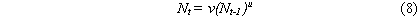where b0 and d0 are certain constants. Inserting (1a) into equation (5) yields: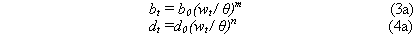where p and q are certain constants. The differential equation (6a) has the time-independent solution Nt = N0 = p 1/η(m – n), which represents a horizontal line. As with the above model, the solution curves that are beneath this line monotonically increase and those lying above the line – monotonically decrease. Thus, this model has the same limitation as Wood's model and its other derivatives: it does not offer oscillating solutions.

Brander and Taylor (1998) have suggested another popular model. This model analyzes some abstract renewable resource consumed in the course of human activities. For example, it might be forest resources or soil yield. St is the available amount of this resource (in year t), and K denotes its reserve in nature. The equation for consumption of this resource is as follows: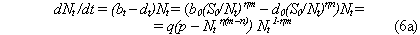where r and u are certain constants. The first term on the right side describes the process of natural resource renewal; the second term describes resource depletion owing to economic activity. The population number is given by the following equation: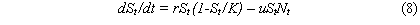where d and v are constants, and d < 0 in this case. This equation shows that natural population growth depends on the availability of resource St.

Brander and Taylor have shown that the system of equations (8)–(9) has oscillating solutions: when the resource is abundant the population grows, when it is exhausted the population decreases until the resource is renewed. Brander and Taylor refer to their model as ‘Malthusian-Ricardian’. Initially, the model was intended to describe the economy of Easter Island, but afterwards it got wider application as a sufficiently general model of the Malthusian economy (e.g., Maxwell and Reuveny 2000; D'Alessandro 2007). It is essential to note, however, that resource St in the Brander–Taylor model is not the harvest gathered by farmers. According to Brander and Taylor, the crop is denoted by the term uStNt and it is deducted from the resource St. According to Szulga (2012), such a model describes a society of gatherers (or hunters) rather than an agrarian society. Meanwhile, Malthus mainly studied agricultural economies. Thus, Brander-Taylor model cannot be referred to as a ‘Malthusian-Ricardian’ one.

Up to this point, I have confined my discussion to the analysis of simple models of the Malthusian economy that consist of no more than two differential equations. Naturally, there exist more complicated models (e.g., Usher 1989; Komlos and Artzrouni 1990; Chu and Lee 1994; Galor and Weil 2000; Lee and Tuljapurkar 2008) that allow for better behavioral freedom and offer oscillating solutions, as well. Many such models have been constructed within the framework of cliodynamic research actively performed in Russia and the USA (e.g., Tsirel 2004; Korotayev, Malkov, and Khaltourina 2005, 2006; Korotayev, Malkov, and Grinin 2007; Turchin 2007, 2009; Malkov 2009). However, almost all models described in literature have the same drawback: they contain uncertain coefficients whose values are unknown and cannot be determined in principle. The more complicated is a model, the more uncertain coefficients it contains. Meanwhile, these coefficients determine the behavior of the model, and different coefficient values result in different population dynamics. Thus, an uncertainty originates: as coefficient values are unknown, it is also uncertain which of the possible behavioral variants corresponds to historical reality and which of them could not possibly be realized.

In the remainder of this article, I would like to discuss two simple models that contain no uncertain parameters and, in my opinion, are sufficiently adequate for the description of the Malthusian population dynamics. Within the first model, Nt is the population in the year t, as above; Kt is the grain stock after the harvest estimated in terms of minimum annual per caput grain consumption rates (rate of consumption is assumed about 240 kg); and r is the natality under favorable conditions. The area under cultivation and the harvest depend on the population, and with the population growth they tend to the constant determined by the maximum area under cultivation maintained by the agricultural community. We will consider that the harvest is determined by the equation Pt=aNt /(Nt+d), where a and d are certain constants. To describe the population dynamics we use the standard logistic equation: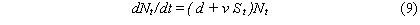Kt in this logistic equation denotes the carrying capacity (i.e., the maximum size of population that may live in this territory). In our case, this population size corresponds to the number of minimum annual consumption rates in storage. Annually, Nt consumption rates are consumed, and the stock growth will be equal to: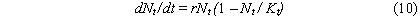Thus, we have the simplest system of two differential equations (10)–(11). This system has an equilibrium state, when the population and stock remain constant, namely, when K0 = = N0 = a – d.

If N in the equation for dP/dN tends to 0, we will obtain the harvest a/d (number of consumption rates) gathered by one farmer in favorable conditions (when the population is small and he or she is able to cultivate the maximum area). Thus, the value q = a/d shows how many households one farming family can support. The history of agricultural societies shows that q usually varies within the limits 1.2 < q < 2. We can express a and d in terms of q and N0:

d = N0 / (q – 1), a = qN0 / (q – 1)

N0 can be conventionally set equal to 1 and thus, there are two constants in this model, r and q, which have physical significance and vary within the known limits: 0.01 < r < 0.02, and 1.2 < q < 2. The common methods used for investigation of dynamic systems allow us to determine that system (10)–(11) describes dying oscillations. The first oscillations can have different periods; however, when the curve approaches the equilibrium state, the period is close to:

T = 2π / √(r – r/q – r2/4)

The period T decreases when r and q increase, and increases, respectively, when these values decrease (Table 1 and Fig. 1).

Table 1

Period of oscillations with various r and q (in years)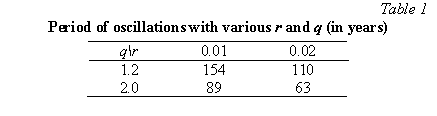Thus, the period of oscillations in this model is comparable to the duration of secular demographic cycles observed in the history of many states (Turchin and Nefedov 2009).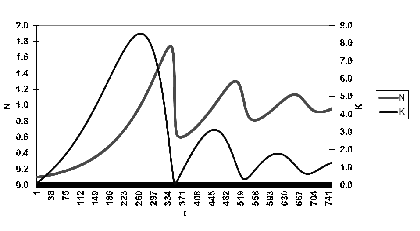Fig. 1. Example of calculations using the model (r = 0.01; p = 1.2)

The dynamics of the agricultural population according to this model have an oscillating nature. In theory these oscillations die out and the system tends to the equilibrium state, but various random impacts and influences neglected herein (e.g., catastrophic crop failure) disturb the system equilibrium, after which there starts a new series of dying oscillations. The peculiar feature of the agricultural society is that its economic dynamics substantially depend on such a random value as the crop yield. The random factors that impact such systems are generally assumed to be exogenous; however, the dependence on crop yield variations is an intrinsic, endogenous feature of agricultural production. Therefore, one arrives at the conclusion that a special random value describing crop yield must be incorporated into the ideal model of the Malthusian cycle. This can be conveniently done within the iterative model where the calculations are made from year to year.

For convenience, I consider production years to start with the harvest, not a specific calendar date. The population number Nt at the beginning of year t is expressed in terms of the number of households or families (conventionally assuming that a household contains five people). In theory (i.e., when there is enough land for cultivation), a farming household cultivates a standard parcel of land (e.g., a Middle Eastern ‘çiftlik’) and one can measure the maximum possible area of arable land in terms of standard parcels S. When the number of households Nt exceeds S, two families can live on some parcels.

Let at represent the annual crop yield t, expressed in terms of minimum family grain consumption rates that can be gathered on a standard parcel. We will express the crop yield in the form at= a0 + + dt, where a0 is the average crop yield, dt is a random value that accepts values from the segment (–а1, а1). The value а1 is less than a0 and the crop yield at varies within the interval of a0 – а1 to a0 + а1. With the units of measure that I have assumed, the harvest Yt (number of consumption rates) can be expressed in the following simple form:

Yt = atNt if Nt < S, and Yt = atS if Nt > S.

If there is a grain surplus in the year t, that is per-capita production yt =Yt/Nt exceeds the ‘satisfactory consumption’ p1 (p1 > 1), then the farmers do not consume all produced grain, but store up the surplus (for the sake of simplicity we will assume that they store half of the surplus). However, it is worth noting that, owing to the storage conditions, the household stock Zt cannot grow to infinity and is limited by certain value Z0. If there are surpluses exceeding this value, they all are consumed. If the year is lean and the production yt falls below the level p1, the farmers take grain from the stock, increasing the consumption, if possible, up to the level p1. If the stock is not sufficient, it is consumed to the full.

The population growth rate rt is determined as the ratio of the population Nt+1 in the following year to the population Nt in the previous year. The growth rate rt depends on the consumption pt. When the consumption is equal to the minimum normal rate (pt =1), the population remains constant (rt = 1). I designate the maximum natural growth r0, and the consumption rate needed to ensure it as p0. I believe that r0 = 1.02, that the maximum annual population growth is two per cent. When 1 < pt < p0, population growth is linearly dependent on consumption, and in the case when pt > p0, it does not increase (r = r0). For pt < 1, the dependence of rt on pt is taken as rt = pt (i.e., in case of crop failure the surviving population will be equal to the number of consumption rates and all people that do not have a sufficient annual food reserve will perish from starvation). Consequently, the population in the following year will be Nt+1 = rt Nt.

Considering the typical case from the Middle East or Russia from the sixteenth to the eighteenth centuries, when every family could obtain two minimal consumption rations from one standard parcel, one can assume a0 = 2 for the numerical experiment. The scatter of crop yield (ratio а1/a0) was large enough (e.g., it was about 60 per cent of average crop yield in Egypt). Hence, it appears that one can assume а1 = 1.2. As for the random value dt , it may be approximated using squared uniform distribution: if w is a value uniformly distributed over the segment (–1.1), then this random value can be taken as dt = а1 w2 sign(w) (Nefedov and Turchin 2007). The maximum number of standard parcels S can be conventionally assumed to equal one million, and the maximum stock to equal ten-year ones (Z0 = 10). Here, I consider a case in which farmers call upon the experience gained by preceding generations and start laying the crop up in storage as soon as the per-capita production exceeds 1.05 of the minimum level (p1 = 1.05). This calculation has an idealized character, allowing one to assume N1 = 0.8 as the initial population value (in year t = 1). As the calculation results depend on a random value (i.e., crop yield), they can vary with each program run. Despite this variation, one can qualitatively observe a pattern of demographic cycles that seems typical: population growth periods alternate with demographic catastrophes. The duration of this cycle is, as in the previous model, 80–150 years (Fig. 2).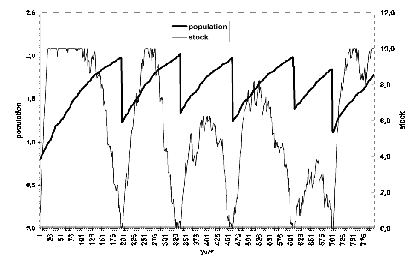Fig. 2. Example of calculation using this model for r0 =1.02, p0 = 2, a0 = 2, а1=1.2, p1=1.05

Naturally, this model describes just the basic mechanism of the demographic cycle omitting many details (e.g., the existence of the state and military elite, the emergence of large landowners). Such factors are taken into account in other models (e.g., Nefedov and Turchin 2007) and the calculations made using these models show that the qualitative cyclic pattern changes insignificantly compared to the suggested model. On the whole, it seems quite certain that the availability of grain stock in farms contributes to a long-term economic stabilization. However, the population growth results in the stock depletion, and, sooner or later, major harvest failures provoke catastrophic starvations followed by events like epidemics, uprisings of starving people, and/or invasions by external enemies seeking to take advantage. As a result, the population size can decrease even by half and a new demographic cycle starts. While the model calculations suggest that this new cycle might start immediately after the catastrophe, in real life such crises as wars and uprisings have some inertia and impede economic revival. In such cases, stabilization is delayed.

Finally, it is worth noting that after the publication of Wood's model economic historians came to consider the Malthusian economy as a system where the population size cannot exceed the carrying capacity and, consequently, the ‘Malthusian crisis’ is impossible. For example, Read and LeBlanc (2003: 59) suggest that

… there is a standard model for the pattern of human population growth and its relationship to carrying capacity (K), namely, that most of the time human populations have low to nonexistent rates of growth… The model is often implicit and may simply assert that, until recently, population sizes have always been well below K and growth rates very low.

But Le Roy Ladurie, Postan, Hatcher, and many other economic historians insist that ‘the Malthusian crises’ were quite common phenomena in lived history, a fact acknowledged by Wood himself. The models described in this article show that the inevitability of similar crises arises from the simple laws that rule the functioning of agrarian economies.

References

Allen, R. 2008. A Review of Gregory Clark's A Farewell to Alms: A Brief Economic History of the World. Journal of Economic Literature 46: 946–973.

Ashraf, Q., and Galor, O. 2011. Dynamics and Stagnation in the Malthusian Epoch. American Economic Review 101(5): 2003–2041.

Brander, J., and Taylor, M. 1998. The Simple Economics of Easter Island: A Ricardo-Malthus Model of Renewable Resource Use. The American Economic Review 88: 119–138.

Chu, C. Y. C., and Lee, R. D. 1994. Famine, Revolt, and the Dynastic Cycle. Journal of Population Economics 7(4): 351–378.

D'Alessandro, S. 2007. Non-linear Dynamics of Population and Natural Resources: The Emergence of Different Patterns of Development. Ecological Economics 62: 473–481.

Galor, O., and Weil, D. N. 2000. Population, Technology, and Growth: From Malthusian Stagnation to the Demographic Transition and Beyond. The American Economic Review 90(4): 806–828.

Komlos, J., and Artzrouni, M. 1990. Mathematical Investigations of the Escape from the Malthusian Trap. Mathematical Population Studies 2: 269–287.

Korotayev, A., Malkov, S., and Grinin, L. (eds.) 2007. History and Mathematics: Analysis and Modeling of Socio-Historical Processes. Moscow: URSS. In Russian (Коротаев А., Малков С., Гринин Л. (ред.) История и Математика: Анализ и моделирование социально-исторических процессов. М.: УРСС).

Korotayev, A., Malkov, A., and Khaltourina, D. 2005. Laws of History. Mathematical Modeling of Historical Macro-Processes: Demography, the Economy, Wars. Moscow: URSS. In Russian (Коротаев А. В., Малков А. С., Халтурина Д. А. Законы истории. Математическое моделирование исторических макропроцессов. Демография, экономика, войны. М.: УРСС).

Korotayev, A., Malkov, A., and Khaltourina, D. 2006. Introduction to Social Macrodynamics: Secular Cycles and Millennial Trends. Moscow: Komkniga.

Le Roy Ladurie, E. 1974. The Peasants of Languedoc. Urbana, IL: University of Illinois Press.

Lee, R. 1980. A Historical Perspective on Economic Aspects of the Population Explosion: The Case of Preindustrial England. In Easterlin, R. A. (ed.), Population and Economic Change in Developing Countries (pp. 517–566). Chicago, IL: University of Chicago Press.

Lee, R., and Anderson, M. 2002. Malthus in State Space: Macro Economic-Demographic Relations in English History, 1540–1870. Journal of Population Economics 15(2): 195–220.

Lee, C. T., and Tuljapurkar, S. 2008. Population and Prehistory I: Food-Dependent Population Growth in Constant Environments. Theoretical Population Biology 73: 473–482.

Malkov, S. 2009. Social Self-Organization and the Historical Process: The Possibilities of Mathematical Modeling. Moscow: URSS. In Russian (Малков С. Ю. Социальная самоорганизация и исторический процесс: Возможности математического моделирования. М.: УРСС).

Malthus, T. R. 1798. An Essay on the Principle of Population. London: J. Johnson.

Maxwell, J., and Reuveny, R. 2000. Resource Scarcity and Conflict in Developing Countries. Journal of Peace Research 37(3): 301–322.

Møller, N., and Sharp, P. 2009. Malthus in Cointegration Space: A New Look at Living Standards and Population in Pre-industrial England. Discussion Paper No. 08-16. Copenhagen: Department of Economics, University of Copenhagen.

Nefedov, S. A., and Turchin, P. V. 2007. The Experience of Modeling Demographic and Structural Cycles. In Malkov S., Grinin L., and Korotayev A. (eds.), History and Mathematics: Macro-Historical Dynamics of Society and the State (рр. 153–167). Moscow: URSS. In Russian (Нефедов, С. А., Турчин, П. В. Опыт моделирования демографически-структурных циклов. В Малков, С. Ю., Гринин, Л. Е., Коротаев А. В. (ред.) История и математика: Макроисторическая динамика общества и государства, c. 153–167. М.: УРСС).

Postan, M. M., and Hatcher, J. 1985. Population and Class Relations in Feudal Society. In Aston, T., and Philpin, C. H. E. (eds.), The Brenner Debate: Agrarian Class Structure and Economic Development in Pre-Industrial Europe (pp. 65–78). Cambridge: Cambridge University Press.

Read, D. W., and LeBlanc, S. A. 2003. Population Growth, Carrying Capacity, and Conflict. Current Anthropology 44(1): 59–85.

Szulga, R. S. 2012. Endogenous Population and Resource Cycles in Historical Hunter-Gatherer Economies. Cliodynamics 3(2).

Tsirel, S. V. 2004. On the Possible Reasons for the Hyperexponential Growth of the Earth Population. In Dmitriev, M., and Petrov, A. (eds.), Mathematical Modeling of Social and Economic Dynamics (pр. 367–369). Moscow: Russian State Social University.

Turchin, P. 2007. Historical Dynamics: Towards a Theoretical History. Moscow: LKI. In Russian (Турчин, Р. Историческая динамика. На пути к теоретической истории. М.: ЛКИ).

Turchin, P. 2009. Long-Term Population Cycles in Human Societies. Annals of the New York Academy of Science 1162: 1–17.

Turchin, P., and Nefedov, S. A. 2009. Secular Cycles. Princeton, NJ: Princeton University Press.

Usher, D. 1989. The Dynastic Cycle and the Stationary State. The American Economic Review 79(5): 1031–1044.

Voigtlander, N., and Voth, H. J. 2009. Malthusian Dynamism and the Rise of Europe: Make War, not Love. American Economic Review: Papers & Proceedings 99(2): 248–254.

Wood, J. W. 1998. A Theory of Preindustrial Population Dynamics, Demography, Economy, and Well-being in Malthusian Systems. Current Anthropology 39: 99–135.

10.08.2023

09.08.2023

20.04.2023